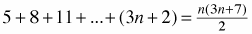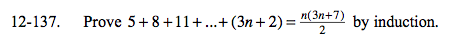### Home > A2C > Chapter 12 > Lesson 12.3.1 > Problem12-137

12-137.

Proveby induction. Homework Help ✎Verify that it is true for n = 1.

$5=\frac{(1)(3(1)+7)}{2}$

5 = 5

Assume that the identity is true for some arbitrary number k.

Prove that given the above assumption, the identity holds true for (k + 1).